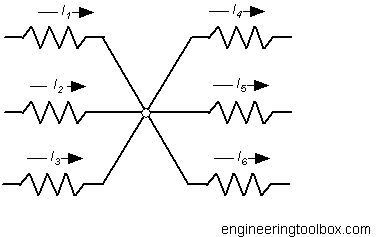Engineering ToolBox - Resources, Tools and Basic Information for Engineering and Design of Technical Applications!

Kirchhoff's Voltage and Current Laws

Kirchhoff's current and voltage laws.

Kirchhoff's Current Law

Also called Kirchhoff's first law, Kirchhoff's point rule, Kirchhoff's junction rule (or nodal rule) or Kirchhoff's first rule.At any junction in an electric circuit the total current flowing towards the junction is equal to the total current flowing away from the junction.

Kirchhoff's Current Law can be expressed as

Σ I = I1 + I2 + .. + In = 0              (1)

where

I = currents (amps)

In general - current toward the junction is positive and current flowing away from the junction is negative.

Example - Kirchhoff's Current Law

The currents in the figure above is I1 = 0.1 A, I2 = 0.2 A, I3 = 0.1 A, I4 = - 0.05 A, I5 = -0.15 A.

I6 can be calculated by using Kirchhoff's current law

(0.1 A) + (0.2 A) + (0.1 A) + (- 0.05 A) + (-0.15 A) + I6 = 0

or

I6 = -((0.1 A) + (0.2 A) + (0.1 A) + (- 0.05 A) + (-0.15 A))

= -0.2 A

Kirchhoff's Voltage Law

Also called Kirchhoff's second law, Kirchhoff's loop (or mesh) rule, or Kirchhoff's second rule.

In a closed loop in a network the sum of the voltage drops or potential differences around the loop is zero.

Kirchhoff's Voltage Law can be expressed as

Σ U = U1 + U2 + .. + Un = 0                       (2)

where

U = voltage drop or potential difference (volts)

In a schematic diagram voltage can be measured positive clockwise or anticlockwise.

Related Topics

• Electrical - Electrical units, amps and electrical wiring, wire gauge and AWG, electrical formulas and motors

Engineering ToolBox - SketchUp Extension - Online 3D modeling!

Add standard and customized parametric components - like flange beams, lumbers, piping, stairs and more - to your Sketchup model with the Engineering ToolBox - SketchUp Extension - enabled for use with the amazing, fun and free SketchUp Make and SketchUp Pro .Add the Engineering ToolBox extension to your SketchUp from the SketchUp Pro Sketchup Extension Warehouse!

Translate

Privacy

We don't collect information from our users. Only emails and answers are saved in our archive. Cookies are only used in the browser to improve user experience.

Some of our calculators and applications let you save application data to your local computer. These applications will - due to browser restrictions - send data between your browser and our server. We don't save this data.

Citation

• Engineering ToolBox, (2008). Kirchhoff's Voltage and Current Laws . [online] Available at: https://www.engineeringtoolbox.com/kirchhoff-laws-d_1386.html [Accessed Day Mo. Year].

Modify access date.

. .

Scientific Online Calculator1 14